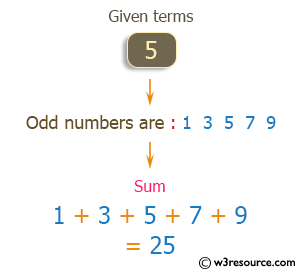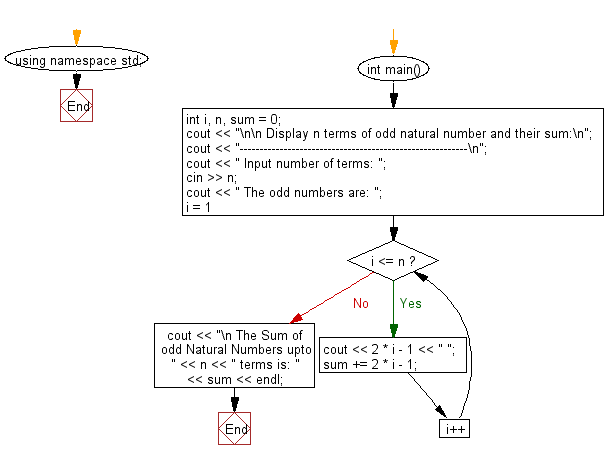﻿ C++ : Display the n terms of odd natural number, their sum# C++ Exercises: Display the n terms of odd natural number and their sum

## C++ For Loop: Exercise-20 with Solution

Write a program in C++ to display the n terms of odd natural number and their sum.

Pictorial Presentation:Sample Solution:-

C++ Code :

``````#include <iostream>
using namespace std;

int main()
{
int i, n, sum = 0;

cout << "\n\n Display n terms of odd natural number and their sum:\n";
cout << "---------------------------------------------------------\n";
cout << " Input number of terms: ";
cin >> n;
cout << " The odd numbers are: ";
for (i = 1; i <= n; i++)
{
cout << 2 * i - 1 << " ";
sum += 2 * i - 1;
}
cout << "\n The Sum of odd Natural Numbers upto " << n << " terms";

" << sum << endl";
}
``````

Sample Output:

```Display n terms of odd natural number and their sum:
---------------------------------------------------------
Input number of terms: 5
The odd numbers are: 1 3 5 7 9
```

Flowchart:C++ Code Editor:

Contribute your code and comments through Disqus.

What is the difficulty level of this exercise?

﻿

## C++ Programming: Tips of the Day

What is a smart pointer and when should I use one?

This answer is rather old, and so describes what was 'good' at the time, which was smart pointers provided by the Boost library. Since C++11, the standard library has provided sufficient smart pointers types, and so you should favour the use of std::unique_ptr, std::shared_ptr and std::weak_ptr.

There was also std::auto_ptr. It was very much like a scoped pointer, except that it also had the "special" dangerous ability to be copied - which also unexpectedly transfers ownership.

It was deprecated in C++11 and removed in C++17, so you shouldn't use it.

```std::auto_ptr<MyObject> p1 (new MyObject());
std::auto_ptr<MyObject> p2 = p1; // Copy and transfer ownership.
// p1 gets set to empty!
p2->DoSomething(); // Works.
p1->DoSomething(); // Oh oh. Hopefully raises some NULL pointer exception.
```

Ref : https://bit.ly/3mc9GHE